Courses

# Thermodynamic Potential MCQ Level – 1

## 10 Questions MCQ Test Topic wise Tests for IIT JAM Physics | Thermodynamic Potential MCQ Level – 1

Description
This mock test of Thermodynamic Potential MCQ Level – 1 for IIT JAM helps you for every IIT JAM entrance exam. This contains 10 Multiple Choice Questions for IIT JAM Thermodynamic Potential MCQ Level – 1 (mcq) to study with solutions a complete question bank. The solved questions answers in this Thermodynamic Potential MCQ Level – 1 quiz give you a good mix of easy questions and tough questions. IIT JAM students definitely take this Thermodynamic Potential MCQ Level – 1 exercise for a better result in the exam. You can find other Thermodynamic Potential MCQ Level – 1 extra questions, long questions & short questions for IIT JAM on EduRev as well by searching above.
QUESTION: 1

### The thermodynamic relation expressing the change at temperature with volume at constant entropy is Select one:

Solution:

From thermodynamic Maxwell equation :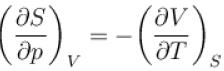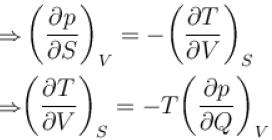The correct answer is: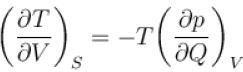QUESTION: 2

### A sample of an ideal gas with initial pressure p and volume V is taken through an isothermal expansion proceed during which the change in entropy is found to be ΔS. The universal gas constant is R. Then, the work done by the gas is given by Select one:

Solution:

By ideal gas equal,
pV = nRT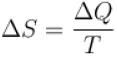Multiplying both the equations,
pVΔS = nRΔQ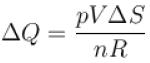∴ For isothermal expansion, ΔT = 0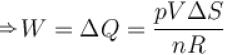QUESTION: 3

### The Gibb’s potential is defined as : Select one:

Solution:

Gibb’s free energy, denoted by G, combines enthalpy and entropy into a single value
The free energy is defined as follows
G = H – TS
Since H = U + pV
G = U + pV – TS
G = U + pV – TS

QUESTION: 4

The thermodynamical relation expressing TdS equation.
Select one:

Solution:

Let the entropy S of a thermodynamic system be a function of temperature T and Volume V i.e.
S = f(T, V)
Since dS is perfect differential, we can write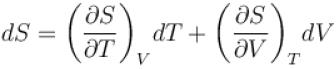Multiplying by T, we get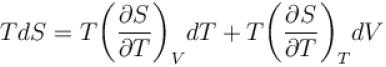For any substance, the specific heat at constant volume is given by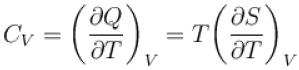Using dQ = TdS
Also, from Maxwell’s equation, we have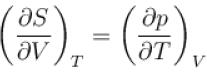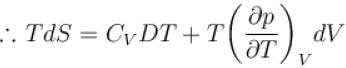The correct answer is: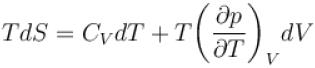QUESTION: 5

The thermodynamical potential enthalpy H = U + pV, then which of the following relation hold true?
Select one:

Solution:

H = U + pV
dH = dU + pdV + Vdp
dH = dQ + Vdp
⇒ dH = TdS + Vdp
Take S to be constant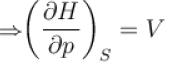take p to be constant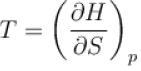The correct answer is: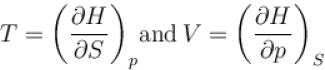QUESTION: 6

The combined form of first and second law of thermodynamics is given by (p = pressure, V = volume, T = temperature, U = internal energy, S = entropy, Q = quantity of heat)
Select one:

Solution:

According to first law of thermodynamics
dQ = dU + pdV ...(1)
According to second law of thermodynamics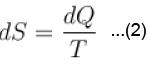Combined form of (1) and (2)
TdS = dU + pdV
The correct answer is: TdS = dU + pdV

QUESTION: 7

Helmholtz free energy in given as F = U – Ts then which of the relations hold true?
Select one:

Solution:

F = U – TS
dF = dU – TdS + SdT
take V to be constant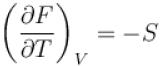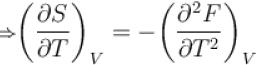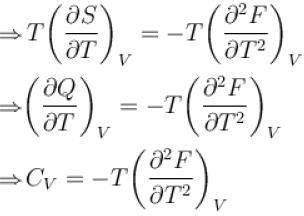The correct answer is: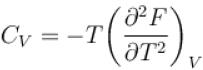QUESTION: 8

The Gibb’s function G in thermodynamics is defined as
G = H – TS
In an isothermal, isobaric, reversible process, G
(where H = Enthalpy, T = Temperature, S = Entropy)
Select one:

Solution:

Condition for a change to occur in any process
dU – TdS ≤ –pdV
d(U – TS + pV) ≤ 0
⇒ d(H – TS) ≤ 0
Since, G = H – TS
We get dG ≤ 0
it means in isothermal, isobaric and reversible process,
dG = 0 or G = constant
The correct answer is: Remains constant but not zero

QUESTION: 9

Gibb’s thermodynamical potential can be represented as G = H – TS. Which relation hold true?
[Where H = Enthalpy, S = Entropy]
Select one:

Solution:

We know that
dU = TdS – pdV + µdN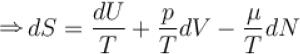take U to be constant and N to be constant
we get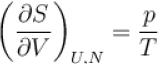The correct answer is: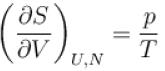QUESTION: 10

The enthalpy of unit mass for any system is
Select one:

Solution:

The enthalpy of a homogeneous system is defined as
H = U + pV
The U term can be interpreted as the energy required to create the system, and the pV terms as the energy that would be required to “make room” for the system if pressure of the environment remained constant.